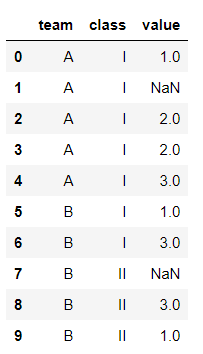# Best way to Impute categorical data using GroupbyWe know that we can replace the nan values with mean or median using fillna(). What if the NAN data is correlated to another categorical column?

We know that we can replace the nan values with mean or median using fillna(). What if the NAN data is correlated to another categorical column?

What if the expected NAN value is a categorical value?

Below are some useful tips to handle NAN values.

Definitely you are doing it with Pandas and Numpy.

``````import pandas as pd
import numpy as np``````

## ngroup

``````cl = pd.DataFrame({
'team':['A','A','A','A','A','B','B','B','B','B'],                   'class'['I','I','I','I','I','I','I','II','II','II'],
'value': [1, np.nan, 2, 2, 3, 1, 3, np.nan, 3,1]})``````Lets assume if you have to fillna for the data of liquor consumption rate, you can just fillna if no other data is relevant to it.

But if the age of the person is given then you can see a pattern in the age and consumption rate variables. Because the liquor consumption will not be in same level for all the people.

An another example is fillna in salary value could be related with age, job title and/or education.

In the above example, let assume that columns test and class are related to value.

Using ngroup you can name the group with the index.

## Authentication In MEAN Stack - A Quick Guide

Everything you should know about Authenticating in MEAN stack applications with comprehensive explanation and necessary code snippets.

## Python Pandas Objects - Pandas Series and Pandas Dataframe

In this post, we will learn about pandas’ data structures/objects. Pandas provide two type of data structures:- ### Pandas Series Pandas Series is a one dimensional indexed data, which can hold datatypes like integer, string, boolean, float...

## What Is MEAN STACK and Scope of MEAN STACK Developer ?

Best Mean Stack training skills from top-rated web development experts. CETPA Offers online basic to advanced courses to help you master the full MEAN Stack including MongoDb, ExpressJS, AngularJS and NodeJs.

## Pandas mean: How to Find Mean in Pandas DataFrame

To find mean of DataFrame, use Pandas mean() function. The DataFrame.mean() function returns the mean of the values for the requested axis.

## Pandas in Python

Pandas is a fast, powerful, flexible and easy to use open source data analysis and manipulation tool, built on top of the Python programming language.